# Bihar Board Class 10th Maths Solutions Chapter 14 Statistics Ex 14.3

Bihar Board Class 10th Maths Solutions Chapter 14 Statistics Ex 14.3 Textbook Questions and Answers.

## BSEB Bihar Board Class 10th Maths Solutions Chapter 14 Statistics Ex 14.3Question 1.
The following frequency distribution gives the monthly consumption of electricity of 68 consumers of a locality. Find the median, mean and mode of the data and compare them.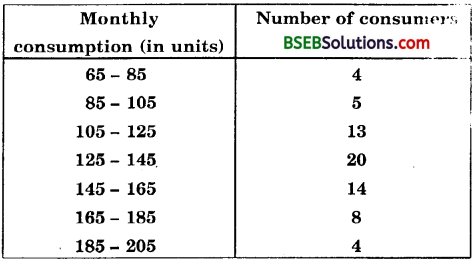Calculation of median
First, we prepare the table given below to compute the median:We have : n = 68 Now, $$\frac { n }{ 2 }$$ = $$\frac { 68 }{ 2 }$$ = 34
The cumulative frequency, just greater than $$\frac { n }{ 2 }$$, is 42 and the corresponding class is 125 – 145. Thus, 125 – 145 is the median class such that
$$\frac { n }{ 2 }$$ = 34, l = 125, c.f. = 22, f= 20 and h = 20.
Substituting these values in the formula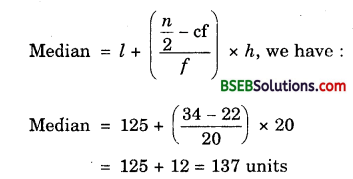Calculation of mean
Let the assumed mean, A = 135 and class size, h = 20.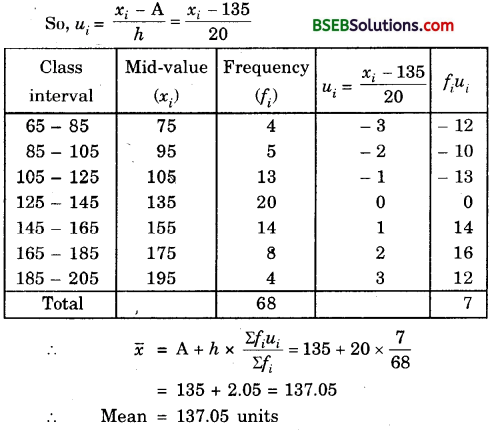Calculation of mode:
The class 125-145 has the maximum frequency. Therefore this is the modal class.
Here, l = 125, h = 20, f1 = 20 f0 = 13 and f2 = 14.
Now, let us substitute these values in the formula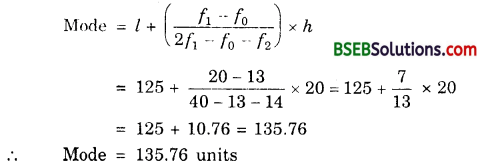Clearly, the three measures are approximately the same in this case.

Question 2.
If the median of the distribution given below is 28.5, find the values pf x and y.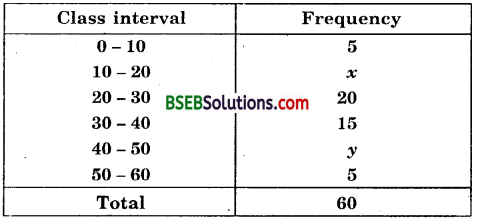Solution:
Here, it is given that median is 28.5.
and n = ∑fi = 60
We now prepare the following cumulative frequency table :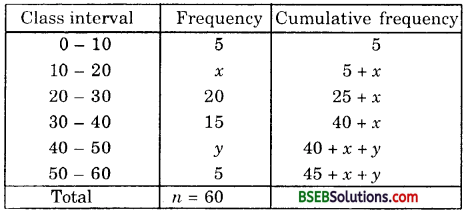Here n = 60. So, $$\frac { n }{ 2 }$$ = 30
Since the median is given to be 28.5, thus the median class is 20 – 30.
∴ l = 20, h = 10, f= 20 and cf = 5 + x
∴ Median = l + $$\left(\frac{\frac{n}{2}-\mathrm{cf}}{f}\right)$$ x h
or 28.5 = 20 + $$\frac{30-(5+x)}{20}$$ x 10
or 28.5 = 20 + $$\frac{25-x}{2}$$
or 57 = 40 + 25 – x
or x – 65 – 57 = 8
Also, 45 + x + y = 60
So, 45 + 8+y = 60 [∵ x = 8]
or y = 60 – 53 = 7
Hence, x = 8 and y = 7.Question 3.
A life insurance agent found the following data for distribution of ages of 100 policy holders. Calculate the median age, if policies are given only to persons having age 18 years onwards but less than 60 year.Solution:
We are given the cumulative frequency distribution. So, we first construct a frequency table from the given cumulative frequency distribution and then we will make necessary computations to compute median.Here, n = ∑fi = 100 So, $$\frac { n }{ 2 }$$ = 50.
We see that the cumulative frequency just greater than $$\frac { n }{ 2 }$$, i.e., 50 is 78 and the corresponding class is 35 35 – 40 is the median class.
∴ $$\frac { n }{ 2 }$$ = 50, l = 35, cf = 45, f = 33 and h = 5.
Now, let us substitute these values in the formulaHence, the median age is 35.76 years.

Question 4.
The lengths of 40 leaves of a plant are measured correct to the nearest millimetre, and the data obtained is represented in the following table :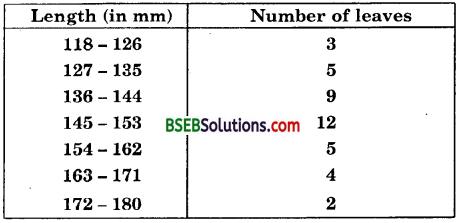Find the median length of the leaves.
Solution:
Here the frequency table is given in the inclusive form. So, we first convert it into exclusive form by subtracting and adding $$\frac { n }{ 2 }$$ to the lower and upper limits respectively of each class, where h denotes the difference of lower limit of a class and the upper limit of the previous class. Converting the given table into exclusive form and preparing the cumulative frequency table, we get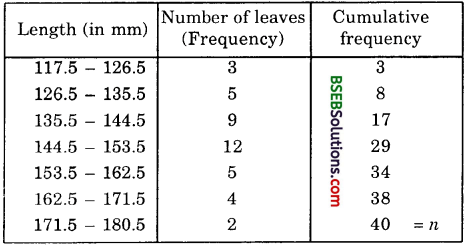We have : n = 40 So, $$\frac { n }{ 2 }$$ = 20.
The cumulative frequency just greater than $$\frac { n }{ 2 }$$ is 29 and the corresponding class is 144.5 – 153.5.
So, 144.5 – 153.5 is the median class.
Here $$\frac { n }{ 2 }$$ = 20, l = 144.5, h = 9, f= 12 and cf = 17.
Substituting these values in the formula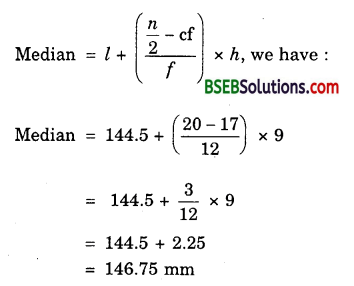Question 5.
The following table gives the distribution of the life time of 400 neon lamps :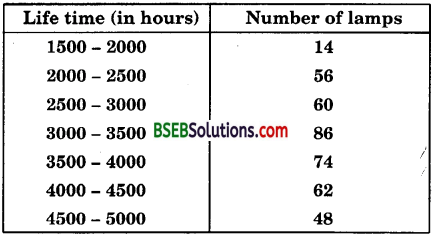Find the median life time of a lamp.
Solution:
First, we prepare the following table to compute the median :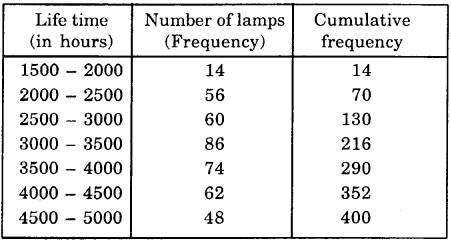We have : n = 400 So, $$\frac { n }{ 2 }$$ = 200.
The cumulative frequency just greater than $$\frac { n }{ 2 }$$ is 216 and the corresponding class is 3000 – 3500. Thus, 3000 – 3500 is the median class such that $$\frac { n }{ 2 }$$ = 200, l = 3000, cf = 130, f = 86 and h = 500.
Substituting these values in the formulaHence, median life time = 3406.98 hoursQuestion 6.
100 surnames were randomly picked up from a local telephone directory and the frequency distribution of the number of letters in English alphabets in the surnames was obtained as follows :Determine the median number of letters in the surnames. Find the mean number of letters in the surnames. Also, find the modal size of the surnames.
[Annual Paper {Delhi) 2008]
Solution:
Calculation of median
First, we prepare the following table to compute the median :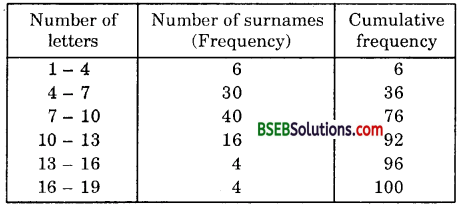We have : n = 100. So, $$\frac { n }{ 2 }$$ = 50.
The cumulative frequency just greater than $$\frac { n }{ 2 }$$ is 76 and the corresponding class is 7 – 10. Thus, 7 – 10 is the median class such that
$$\frac { n }{ 2 }$$ = 50, l = 7, cf = 36, f = 40 and h = 3.
Substituting these values in the formula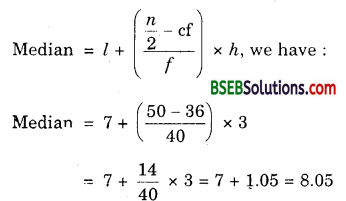Calculation of mean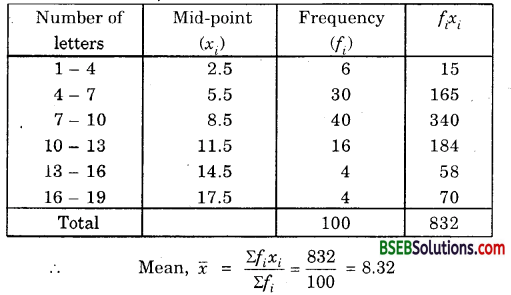Calculation of mode.
The class 7 – 10 has the maximum frequency. Therefore this is the modal class.
Here, 1 = 1, h = 3, f1 = 40, f0 = 30 and f2 = 16.
Now, let us substitute these values in the formulaHence, median = 8.05, mean = 8.32 and mode = 7.88.

Question 7.
The distribution below gives the weights of 30 students of a class. Find the median weight of the students.Solution:
Let us prepare the following table to compute the median :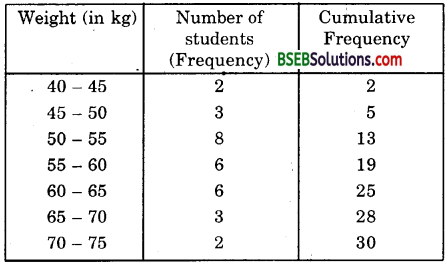We have : n = 30. So, $$\frac { n }{ 2 }$$ = 15
The cumulative frequency just greater than $$\frac { n }{ 2 }$$ is 19 and the corresponding class is 55 – 60.
Thus, 55 – 60 is the median class such that $$\frac { n }{ 2 }$$ = 15, l = 55, f = 6, cf = 13 and h = 5.
Substituting these values in the formulaHence, the median weight is 56.67 kg.